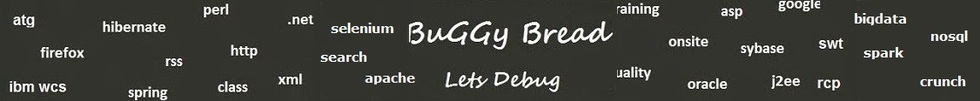Search Java Classes and Packages

Search Java Frameworks and Libraries

255581 classes and counting ...
 Search Tips Index Status

#Agi.foundation.geometry.discrete Classes and Interfaces - 23 results found.
 Name Description Type Package Framework BoxTriangulator Triangulates a box. Class agi.foundation.geometry.discrete AGI ( Analytical Graphics ) CylinderFill Cylinder faces that can be filled. Class agi.foundation.geometry.discrete AGI ( Analytical Graphics ) CylinderTriangulator Triangulates a cylinder. Class agi.foundation.geometry.discrete AGI ( Analytical Graphics ) EllipsoidGrid This class provides a base class for a coverage grid which creates a list of grid points to be used in computing coverage over a region. Class agi.foundation.geometry.discrete AGI ( Analytical Graphics ) EllipsoidGridGlobal This class represents a set of grid points spanning the entire globe. Class agi.foundation.geometry.discrete AGI ( Analytical Graphics ) EllipsoidGridIntersection This class represents a set of grid points created by a given EllipsoidGrid and lying inside a set of EllipsoidSurfaceRegions. Class agi.foundation.geometry.discrete AGI ( Analytical Graphics ) EllipsoidGridLatitudeBounds This class represents a set of grid points bounded by two lines of planetodetic latitude. Class agi.foundation.geometry.discrete AGI ( Analytical Graphics ) EllipsoidGridLatitudeLine This class represents a set of grid points along a line of planetodetic latitude. Class agi.foundation.geometry.discrete AGI ( Analytical Graphics ) EllipsoidGridLongitudeLine This class represents a set of grid points along a line of longitude. Class agi.foundation.geometry.discrete AGI ( Analytical Graphics ) EllipsoidGridPoint This structure represents a grid point on the surface on an ellipsoid. Class agi.foundation.geometry.discrete AGI ( Analytical Graphics ) EllipsoidGridRegions This class provides a method for generating a regular grid inside the boundaries of a specified set of surface regions, based on a given resolution. Class agi.foundation.geometry.discrete AGI ( Analytical Graphics ) EllipsoidGridSpecified A class which wraps a previously generated set of grid points with their respective boundaries or accepts a specified list of Cartographic grid point locations. Class agi.foundation.geometry.discrete AGI ( Analytical Graphics ) EllipsoidTriangulator Triangulates an ellipsoid. Class agi.foundation.geometry.discrete AGI ( Analytical Graphics ) ExtrudedPolylineTriangulator Triangulates a polyline into an extrusion with bottom and top boundaries. Class agi.foundation.geometry.discrete AGI ( Analytical Graphics ) ExtrudedPolylineTriangulatorResult The result from extruded polyline triangulation: a triangle mesh defined using an indexed triangle list with top and bottom boundary positions. Class agi.foundation.geometry.discrete AGI ( Analytical Graphics ) SolidTriangulatorResult The result from a triangulation of a solid: a triangle mesh defined using an indexed triangle list and positions outlining the solid. Class agi.foundation.geometry.discrete AGI ( Analytical Graphics ) SurfaceExtentTriangulator Triangulates an extent on a central body into a triangle mesh and a surrounding boundary. Class agi.foundation.geometry.discrete AGI ( Analytical Graphics ) SurfacePolygonTriangulator Triangulates a polygon, with an optional hole, on a central body, into a triangle mesh and a surrounding boundary. Class agi.foundation.geometry.discrete AGI ( Analytical Graphics ) SurfaceShapes Computes boundary positions for shapes on the surface such as circles, ellipses, and sectors. Class agi.foundation.geometry.discrete AGI ( Analytical Graphics ) SurfaceShapesResult Represents the boundary positions of a shape on the surface computed from by a SurfaceShapes method. Class agi.foundation.geometry.discrete AGI ( Analytical Graphics ) SurfaceTriangulatorResult The result from a triangulation on the surface of a central body: a triangle mesh defined using an indexed triangle list and boundary Class agi.foundation.geometry.discrete AGI ( Analytical Graphics ) TriangulatorResult The result from triangulation: a triangle mesh defined using an indexed triangle list. Class agi.foundation.geometry.discrete AGI ( Analytical Graphics ) WindingOrder Specifies the order for positions or front facing triangles. Class agi.foundation.geometry.discrete AGI ( Analytical Graphics )